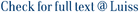We study a problem of optimal investment/consumption over an infinite horizon in a market with two possibly correlated assets: one liquid and one illiquid. The liquid asset is observed and can be traded continuously, while the illiquid one can be traded only at discrete random times, corresponding to the jumps of a Poisson process with intensity λ, is observed at the trading dates, and is partially observed between two different trading dates. The problem is a nonstandard mixed discrete/continuous optimal control problem, which we solve by a dynamic programming approach. When the utility has a general form, we prove that the value function is the unique viscosity solution of the associated Hamilton–Jacobi–Bellman equation and characterize the optimal allocation in the illiquid asset. In the case of power utility, we establish the regularity of the value function needed to prove the verification theorem, providing the complete theoretical solution of the problem. This enables us to perform numerical simulations, so as to analyze the impact of time illiquidity and how this impact is affected by the degree of observation.

Impact of time illiquidity in a mixed market without full observation / Federico, Salvatore; Gassiat, Paul; Gozzi, Fausto. - In: MATHEMATICAL FINANCE. - ISSN 0960-1627. - 27:2(2017), pp. 401-437. [10.1111/mafi.12101]

### Impact of time illiquidity in a mixed market without full observation

#### Abstract

We study a problem of optimal investment/consumption over an infinite horizon in a market with two possibly correlated assets: one liquid and one illiquid. The liquid asset is observed and can be traded continuously, while the illiquid one can be traded only at discrete random times, corresponding to the jumps of a Poisson process with intensity λ, is observed at the trading dates, and is partially observed between two different trading dates. The problem is a nonstandard mixed discrete/continuous optimal control problem, which we solve by a dynamic programming approach. When the utility has a general form, we prove that the value function is the unique viscosity solution of the associated Hamilton–Jacobi–Bellman equation and characterize the optimal allocation in the illiquid asset. In the case of power utility, we establish the regularity of the value function needed to prove the verification theorem, providing the complete theoretical solution of the problem. This enables us to perform numerical simulations, so as to analyze the impact of time illiquidity and how this impact is affected by the degree of observation.
##### Scheda breve Scheda completa Scheda completa (DC)Hamilton-Jacobi-Bellman equation; Investment-consumption problem; Liquidity risk; Optimal stochastic control; Regularity of viscosity solutions; Viscosity solutions
Impact of time illiquidity in a mixed market without full observation / Federico, Salvatore; Gassiat, Paul; Gozzi, Fausto. - In: MATHEMATICAL FINANCE. - ISSN 0960-1627. - 27:2(2017), pp. 401-437. [10.1111/mafi.12101]
File in questo prodotto:
File
FGGMAFI2017.pdf

Open Access

Tipologia: Documento in Pre-print
Licenza: DRM non definito
Dimensione 451.43 kB
11385-159899.pdf

Solo gestori archivio

Tipologia: Versione dell'editore
Licenza: DRM non definito
Dimensione 461.99 kB
Utilizza questo identificativo per citare o creare un link a questo documento: `http://hdl.handle.net/11385/159899`
•4
•3